## 【LeetCode题目详解】第八章 贪心算法 part01 理论基础 455.分发饼干 376. 摆动序列 53. 最大子序和 day31补

article2023/10/1 1:50:41/

# 关于贪心算法，你该了解这些！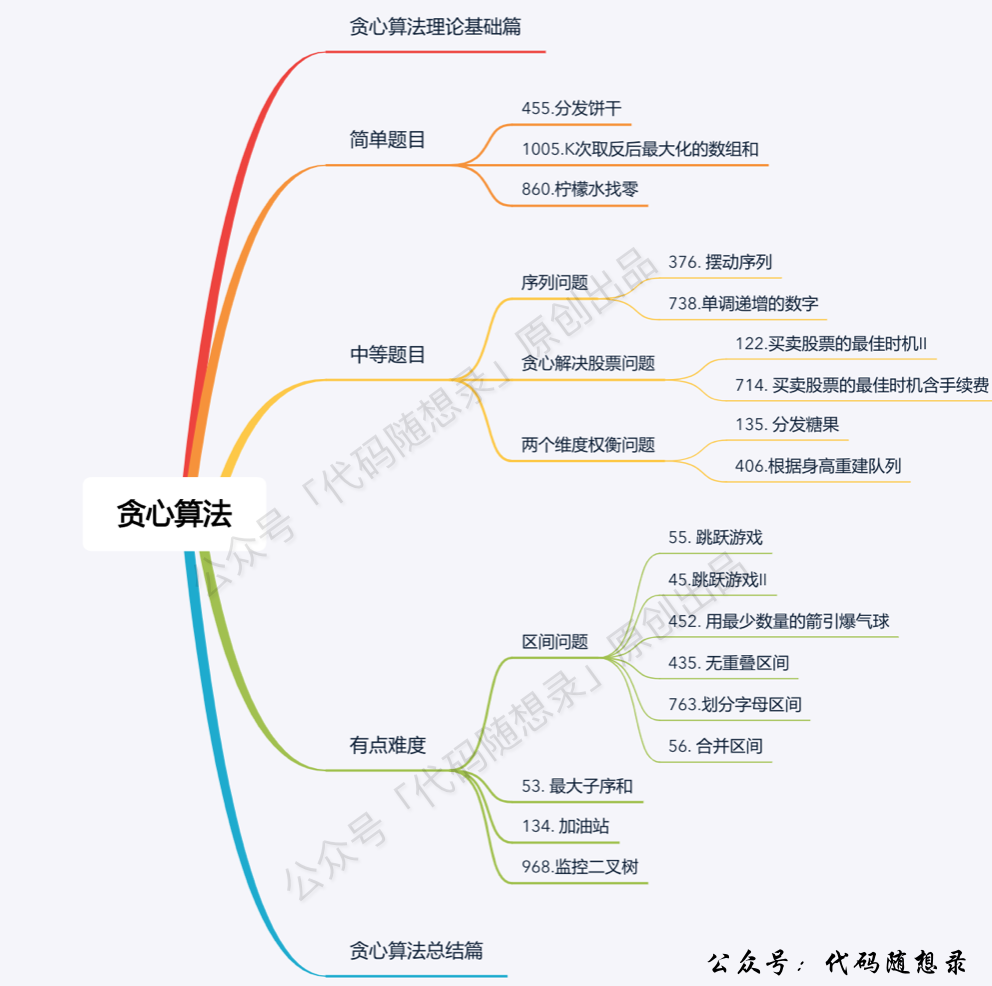## # 贪心的套路（什么时候用贪心）

• 数学归纳法
• 反证法

(opens new window)，这道题不用数学推导一下，就找不出环的起始位置，想试一下就不知道怎么试，这种题目确实需要数学简单推导一下。

## # 贪心一般解题步骤

• 将问题分解为若干个子问题
• 找出适合的贪心策略
• 求解每一个子问题的最优解
• 将局部最优解堆叠成全局最优解

## # 总结# 一、力扣第455题：分发饼干

## 题目:

```输入: g = [1,2,3], s = [1,1]

```

```输入: g = [1,2], s = [1,2,3]

```

• `1 <= g.length <= 3 * 104`
• `0 <= s.length <= 3 * 104`
• `1 <= g[i], s[j] <= 231 - 1`

## 思路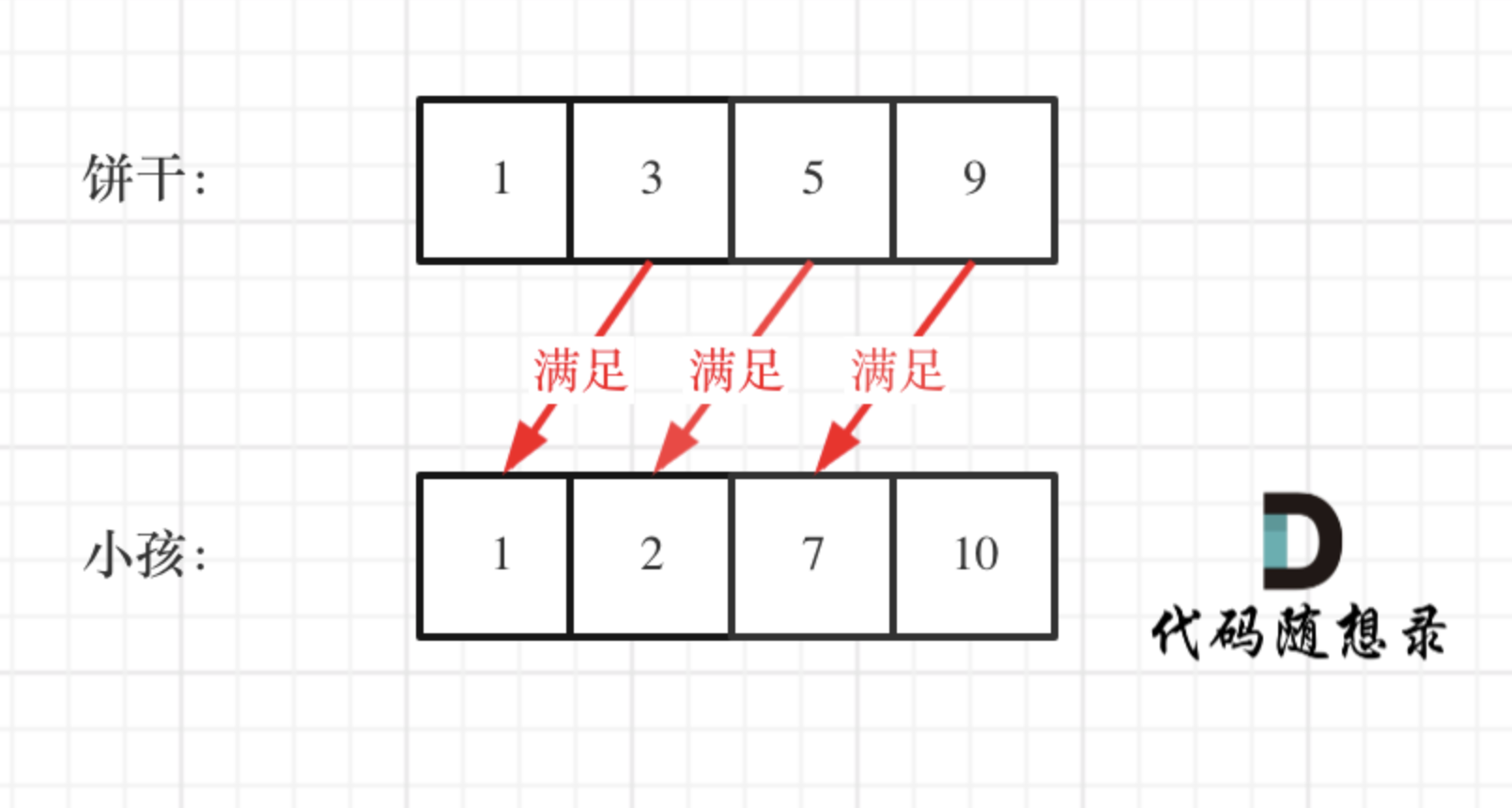C++代码整体如下：

``````// 版本一
class Solution {
public:
int findContentChildren(vector<int>& g, vector<int>& s) {
sort(g.begin(), g.end());
sort(s.begin(), s.end());
int index = s.size() - 1; // 饼干数组的下标
int result = 0;
for (int i = g.size() - 1; i >= 0; i--) { // 遍历胃口
if (index >= 0 && s[index] >= g[i]) { // 遍历饼干
result++;
index--;
}
}
return result;
}
};
``````
• 时间复杂度：O(nlogn)
• 空间复杂度：O(1)

### # 注意事项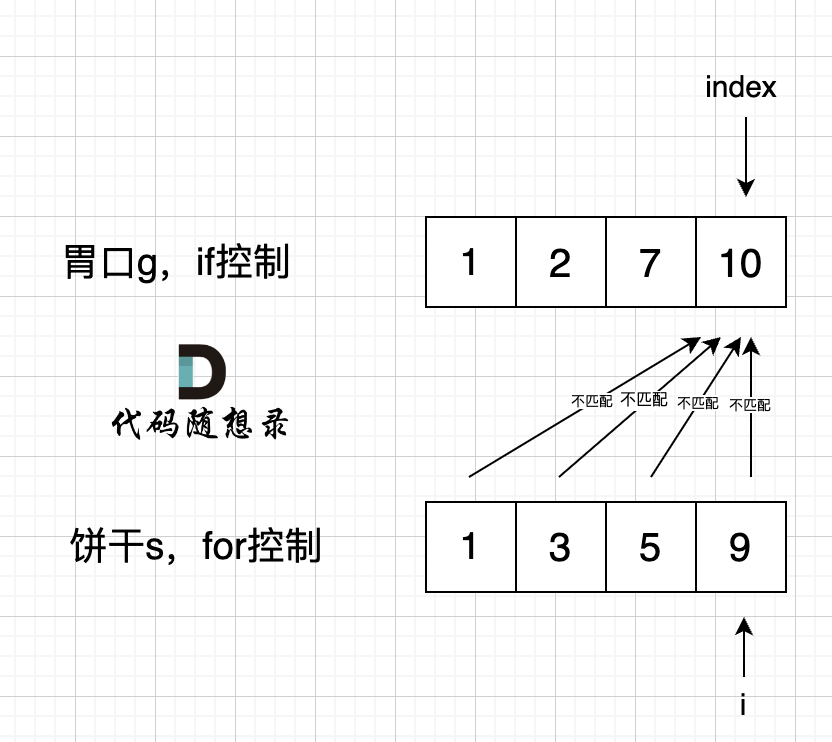if 里的 index 指向 胃口 10， for 里的 i 指向饼干 9，因为 饼干 9 满足不了 胃口 10，所以 i 持续向前移动，而 index 走不到`s[index] >= g[i]` 的逻辑，所以 index 不会移动，那么当 i 持续向前移动，最后所有的饼干都匹配不上。

### # 其他思路

``````class Solution {
public:
int findContentChildren(vector<int>& g, vector<int>& s) {
sort(g.begin(),g.end());
sort(s.begin(),s.end());
int index = 0;
for(int i = 0; i < s.size(); i++) { // 饼干
if(index < g.size() && g[index] <= s[i]){ // 胃口
index++;
}
}
return index;
}
};
``````
• 时间复杂度：O(nlogn)
• 空间复杂度：O(1)

# 二、力扣第376题：摆动序列

## 题目：

• 例如， `[1, 7, 4, 9, 2, 5]` 是一个 摆动序列 ，因为差值 `(6, -3, 5, -7, 3)` 是正负交替出现的。

• 相反，`[1, 4, 7, 2, 5]` 和 `[1, 7, 4, 5, 5]` 不是摆动序列，第一个序列是因为它的前两个差值都是正数，第二个序列是因为它的最后一个差值为零。

```输入：nums = [1,7,4,9,2,5]

```

```输入：nums = [1,17,5,10,13,15,10,5,16,8]

```

```输入：nums = [1,2,3,4,5,6,7,8,9]

```

• `1 <= nums.length <= 1000`
• `0 <= nums[i] <= 1000`

## 思路

### # 思路 1（贪心解法）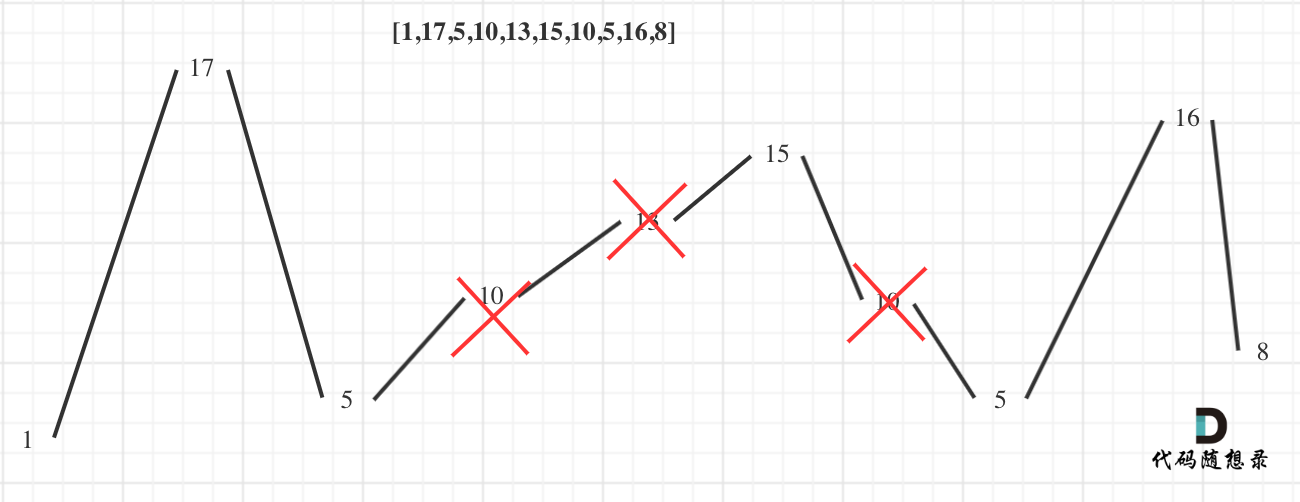（为方便表述，以下说的峰值都是指局部峰值）

1. 情况一：上下坡中有平坡
2. 情况二：数组首尾两端
3. 情况三：单调坡中有平坡

#### # 情况一：上下坡中有平坡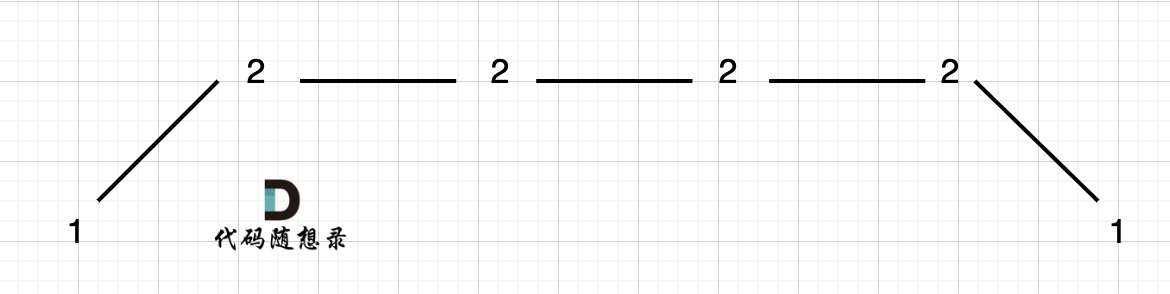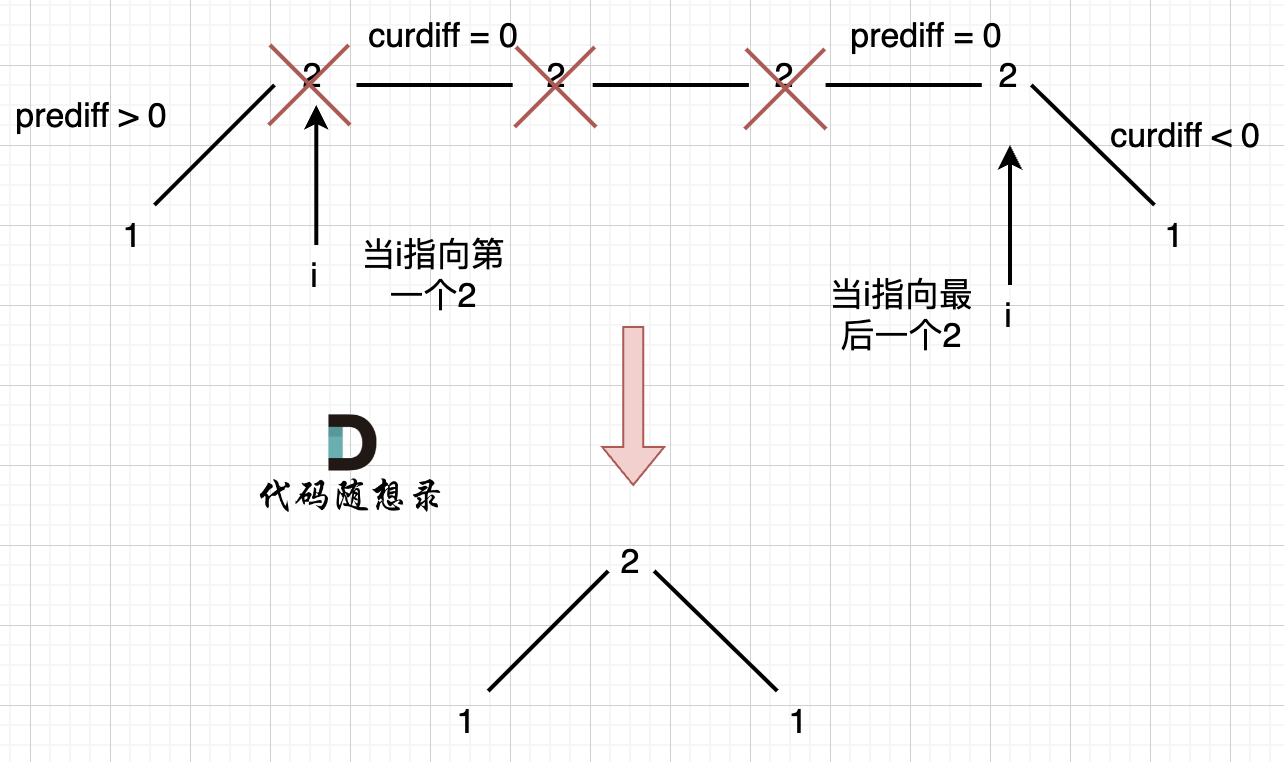#### # 情况二：数组首尾两端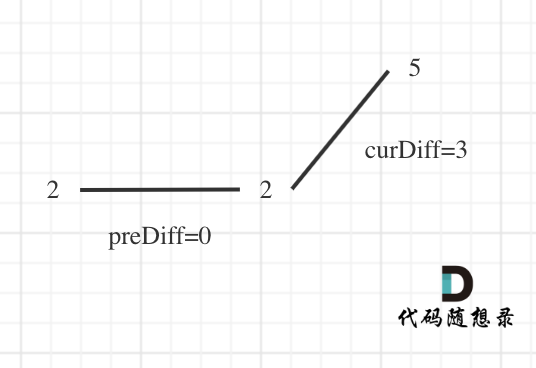``````// 版本一
class Solution {
public:
int wiggleMaxLength(vector<int>& nums) {
if (nums.size() <= 1) return nums.size();
int curDiff = 0; // 当前一对差值
int preDiff = 0; // 前一对差值
int result = 1;  // 记录峰值个数，序列默认序列最右边有一个峰值
for (int i = 0; i < nums.size() - 1; i++) {
curDiff = nums[i + 1] - nums[i];
// 出现峰值
if ((preDiff <= 0 && curDiff > 0) || (preDiff >= 0 && curDiff < 0)) {
result++;
}
preDiff = curDiff;
}
return result;
}
};
``````
• 时间复杂度：O(n)
• 空间复杂度：O(1)

#### # 情况三：单调坡度有平坡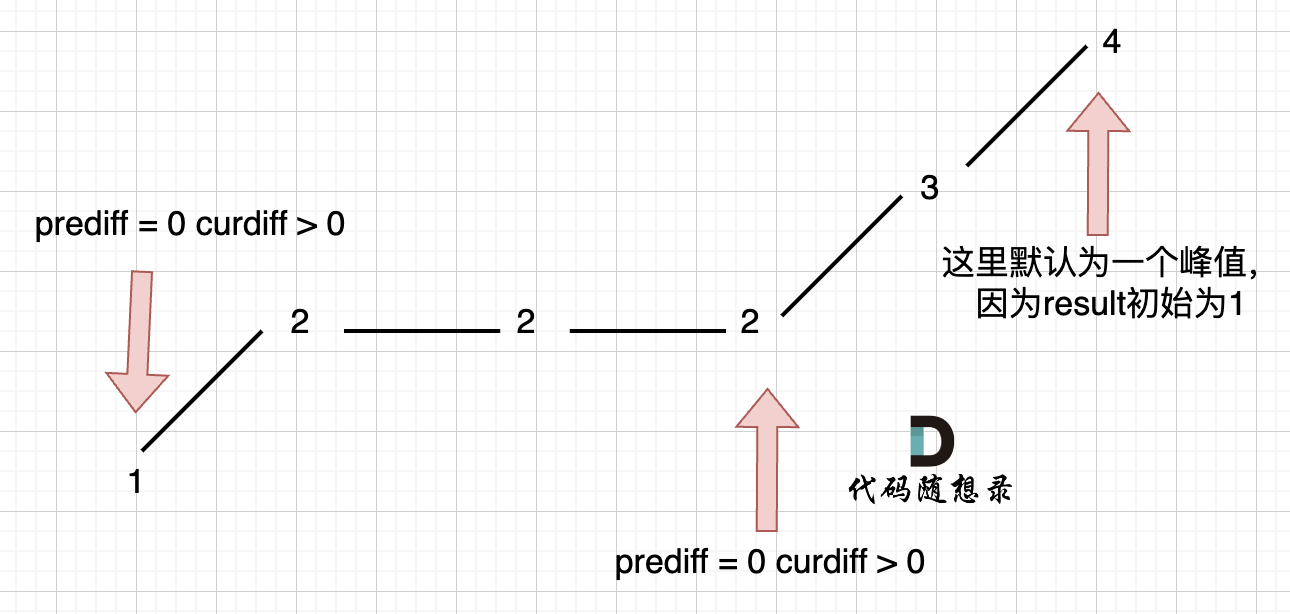``````
// 版本二
class Solution {
public:
int wiggleMaxLength(vector<int>& nums) {
if (nums.size() <= 1) return nums.size();
int curDiff = 0; // 当前一对差值
int preDiff = 0; // 前一对差值
int result = 1;  // 记录峰值个数，序列默认序列最右边有一个峰值
for (int i = 0; i < nums.size() - 1; i++) {
curDiff = nums[i + 1] - nums[i];
// 出现峰值
if ((preDiff <= 0 && curDiff > 0) || (preDiff >= 0 && curDiff < 0)) {
result++;
preDiff = curDiff; // 注意这里，只在摆动变化的时候更新prediff
}
}
return result;
}
};
``````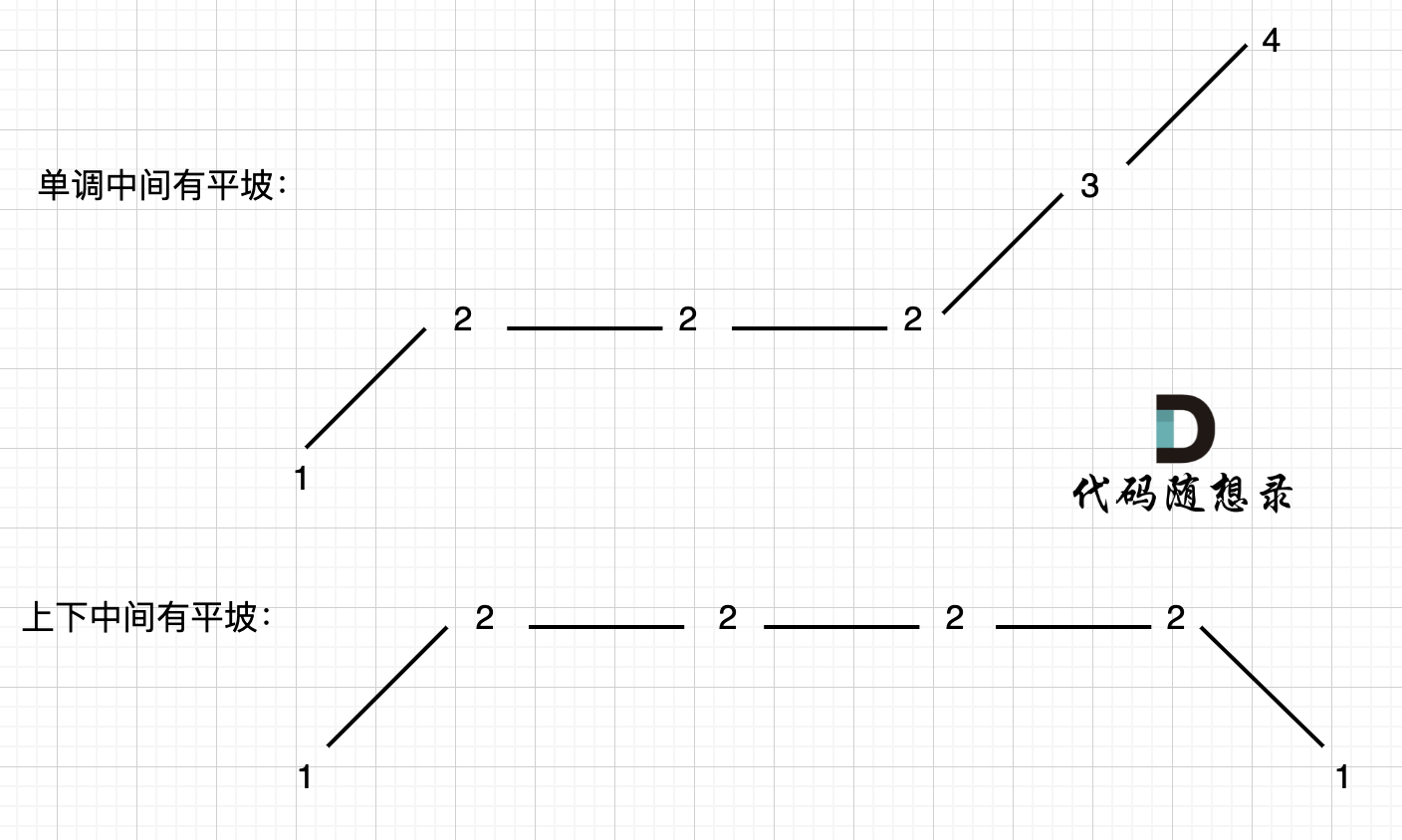### # 思路 2（动态规划）

• 设 dp 状态`dp[i]`，表示考虑前 i 个数，第 i 个数作为山峰的摆动子序列的最长长度
• 设 dp 状态`dp[i]`，表示考虑前 i 个数，第 i 个数作为山谷的摆动子序列的最长长度

• `dp[i] = max(dp[i], dp[j] + 1)`，其中`0 < j < i``nums[j] < nums[i]`，表示将 nums[i]接到前面某个山谷后面，作为山峰。
• `dp[i] = max(dp[i], dp[j] + 1)`，其中`0 < j < i``nums[j] > nums[i]`，表示将 nums[i]接到前面某个山峰后面，作为山谷。

C++代码如下：

``````class Solution {
public:
int dp;
int wiggleMaxLength(vector<int>& nums) {
memset(dp, 0, sizeof dp);
dp = dp = 1;
for (int i = 1; i < nums.size(); ++i) {
dp[i] = dp[i] = 1;
for (int j = 0; j < i; ++j) {
if (nums[j] > nums[i]) dp[i] = max(dp[i], dp[j] + 1);
}
for (int j = 0; j < i; ++j) {
if (nums[j] < nums[i]) dp[i] = max(dp[i], dp[j] + 1);
}
}
return max(dp[nums.size() - 1], dp[nums.size() - 1]);
}
};
``````
• 时间复杂度：O(n^2)
• 空间复杂度：O(n)

• 每次更新`dp[i]`，则在`tree1``nums[i]`位置值更新为`dp[i]`
• 每次更新`dp[i]`，则在`tree2``nums[i]`位置值更新为`dp[i]`
• 则 dp 转移方程中就没有必要 j 从 0 遍历到 i-1，可以直接在线段树中查询指定区间的值即可。

# 三、力扣第53题：最大子数组和

```输入：nums = [-2,1,-3,4,-1,2,1,-5,4]

```

```输入：nums = 

```

```输入：nums = [5,4,-1,7,8]

```

• `1 <= nums.length <= 105`
• `-104 <= nums[i] <= 104`

## 暴力解法

``````class Solution {
public:
int maxSubArray(vector<int>& nums) {
int result = INT32_MIN;
int count = 0;
for (int i = 0; i < nums.size(); i++) { // 设置起始位置
count = 0;
for (int j = i; j < nums.size(); j++) { // 每次从起始位置i开始遍历寻找最大值
count += nums[j];
result = count > result ? count : result;
}
}
return result;
}
};
``````
• 时间复杂度：O(n^2)
• 空间复杂度：O(1)

## # 贪心解法

``````if (count > result) result = count;
``````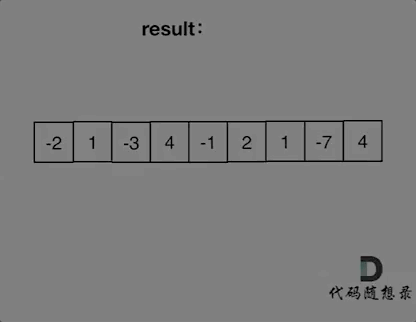``````class Solution {
public:
int maxSubArray(vector<int>& nums) {
int result = INT32_MIN;
int count = 0;
for (int i = 0; i < nums.size(); i++) {
count += nums[i];
if (count > result) { // 取区间累计的最大值（相当于不断确定最大子序终止位置）
result = count;
}
if (count <= 0) count = 0; // 相当于重置最大子序起始位置，因为遇到负数一定是拉低总和
}
return result;
}
};
``````
• 时间复杂度：O(n)
• 空间复杂度：O(1)

## # 动态规划

(opens new window)

``````class Solution {
public:
int maxSubArray(vector<int>& nums) {
if (nums.size() == 0) return 0;
vector<int> dp(nums.size(), 0); // dp[i]表示包括i之前的最大连续子序列和
dp = nums;
int result = dp;
for (int i = 1; i < nums.size(); i++) {
dp[i] = max(dp[i - 1] + nums[i], nums[i]); // 状态转移公式
if (dp[i] > result) result = dp[i]; // result 保存dp[i]的最大值
}
return result;
}
};
``````
• 时间复杂度：O(n)
• 空间复杂度：O(n)

## day31补

### C语言每日一题 ---- 打印从1到最大的n位数（Day 1）

element-ui el-upload组件 on-remove事件 传自定义参数 1.vue页面 :on-remove"(file, fileList) > {handleRemove(file, fileList, item.order)}"2.methods方法里面

### Redis 7 第三讲 数据类型 进阶篇

⑥ *位图 bitmap 1. 理论 由0和1 状态表现的二进制位的bit 数组。 说明：用String 类型作为底层数据结构实现的一种统计二值状态的数据类型 位图本质是数组，它是基于String 数据类型的按位操作。该数组由多个二进制位组成，每个二进制位都对应一个偏…

### clickhouse一次异常排查记录

clickhouse中报错 关闭了自启动&#xff0c;删了status&#xff0c;重启了clickhouse还是报错 1&#xff0c;排查定时执行的脚本日志&#xff08;每小时第5分钟执行&#xff09; INSERT INTO quality0529.previously_reported_urls (url) SELECT url FROM quality0529.hourly_…

### 泡泡玛特回应头部IP营收增速放缓：IP上市时间不固定

8月23日&#xff0c;针对今年上半年头部IP营收增速放缓问题&#xff0c;泡泡玛特&#xff08;09992.HK&#xff09;管理层在业绩会上解释称&#xff0c;每个IP上市时间并不固定&#xff0c;单从上半年看同比增长会有偏差&#xff0c;而随着下半年两个新系列的推出&#xff0c;全…

### FastStone Capture

FastStone Capture 简介下载安装注册 简介 FastStone Capture是一款用于屏幕截图和屏幕录制的工具。它允许用户捕捉屏幕上的内容&#xff0c;并将其保存为图像文件&#xff0c;还可以录制屏幕活动为视频文件。 FastStone Capture官网: https://www.faststone.org/FSCaptureDet…

### -9501 MAL系统没有配置或者服务器不是企业版（dm8达梦数据库）

dm8达梦数据库 -9501 MAL系统没有配置或者服务器不是企业版&#xff09; 环境介绍1 环境检查2 问题原因 环境介绍 搭建主备集群时&#xff0c;遇到报错-9501 MAL系统没有配置或者服务器不是企业版 1 环境检查 检查dmmal.ini配置文件权限正确 dmdba:dinstall&#xff0c;内容正…

### Leetcode刷题笔记--Hot31-40

1--颜色分类&#xff08;75&#xff09; 主要思路&#xff1a; 快排 #include <iostream> #include <vector>class Solution { public:void sortColors(std::vector<int>& nums) {quicksort(nums, 0, nums.size()-1);}void quicksort(std::vector<int…